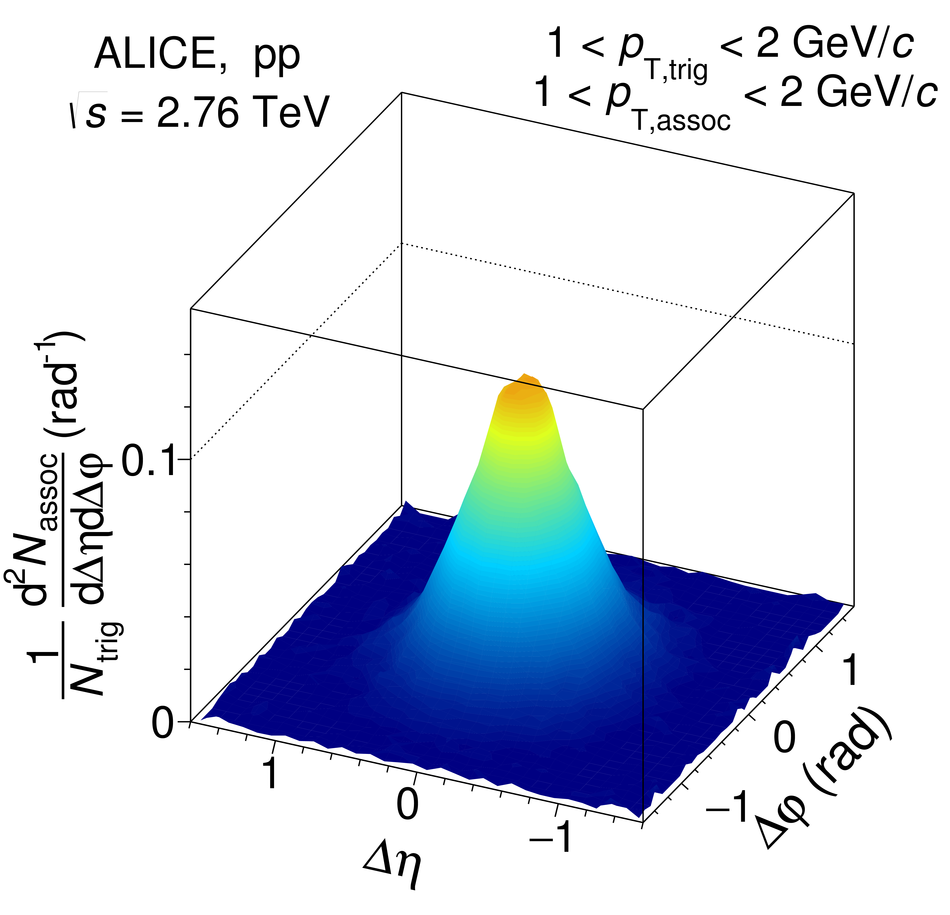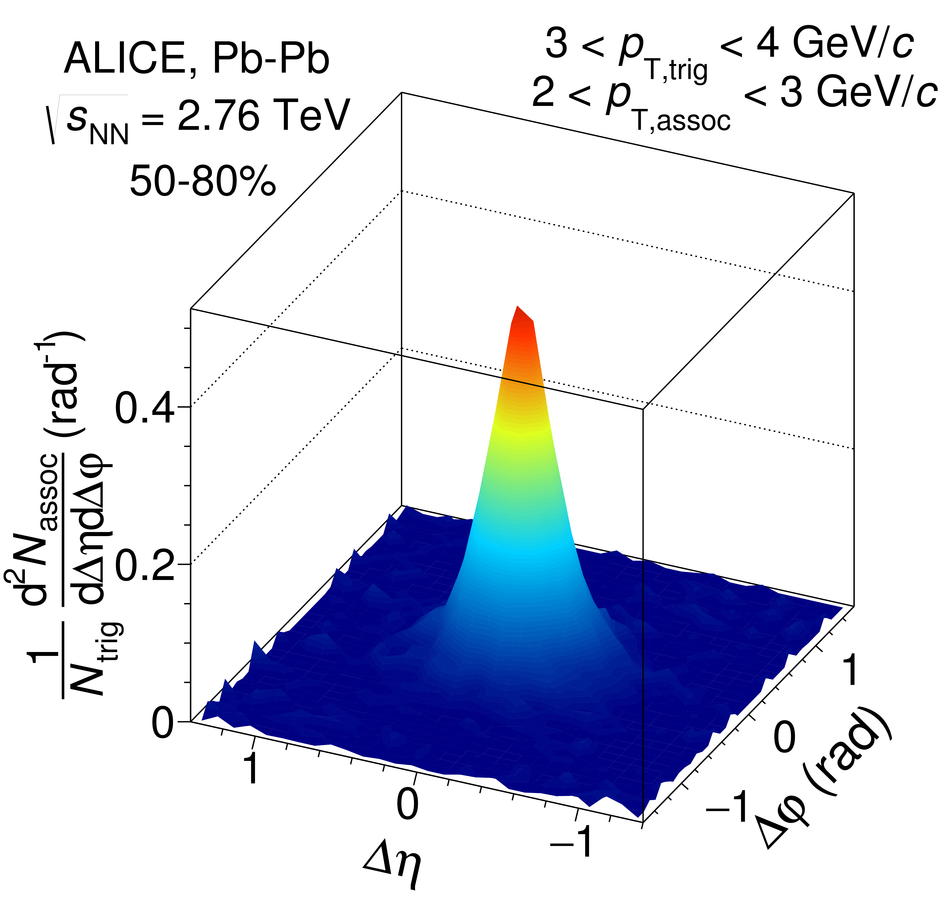# Figure 3

 Associated yield per trigger particle as a function of $\Dphi$ and $\Deta$ in pp collisions (left panels) and \mbox{Pb--Pb} collisions at \snn\ = \unit[2.76]{TeV} in the 50--80\% centrality class (middle panels) and in the 0--10\% centrality class (right panels). The top row shows $1< \pta<$~\unit{\gevc} and $1< \ptt<$~\unit{\gevc} and the bottom row shows $2< \pta<$~\unit{\gevc} and $3< \ptt<$~\unit{\gevc}. The background obtained from the fit function has been subtracted in order to emphasize the near-side peak